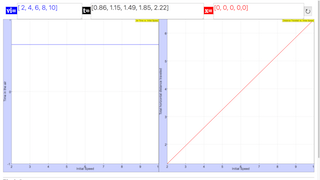Intro Page

Projectile Motion: Experiment and Computational Model

Developed by Todd Zimmerman

Connecting computation to experiment is at the heart of physics. This set of exercises requires students to create a computational model for a ball launched into the air to determine the distance traveled and total time in the air. The students then must perform the experiment by launching the ball with a spring launcher. Data from the experiment must be entered into the computational model and the experimental results are compared to the computer model.

 Subject Areas Mechanics and Experimental Labs First Year IPython/Jupyter Notebook and Sage Worksheet Explain that the time an object is in the air depends only on motion in the y-direction (Exercise 4) Relate the initial velocity of a launched ball to the horizontal velocity when the ball is launched horizontally (Exercise 2) Make use of the fact that horizontal and vertical motion are independent to solve a 2D motion problem by breaking problem up into two 1D problems (Exercise 1) Convert equations of motion into a computational model (program) with discrete time-steps (Exercise 1) Use experimental data as an input into a computational model (Exercise 6) Explain the limitations of computational models in predicting experimental results (Exercise 6) 180 min
Exercise 2

### EXERCISE 2

Predictions

Before you proceed with this part of the exercise make a prediction of how the time the ball takes to hit the floor varies as you increase the initial speed for the case of the ball being launched horizontally. Do you think the ball will be in the air longer, the same, or a smaller time than the initial launch speed?

Make another prediction about how the total horizontal distance the ball travels before hitting the ground as you increase the initial launch speed of the horizontally launched ball. Do you think the horizontal distance will increase, remain the same, or decrease as you increase the initial speed.

Run the Model

Once you’ve made your prediction, run your model with five different initial speeds and record the total horizontal distance and the total time the ball is in the air. Create two graphs from this data, one for horizontal distance vs. initial launch velocity and another graph for total air time vs. initial launch velocity.

How do your predictions compare to the output from the computational model? If your predictions differed from the model results explain how your thoughts have changed as a result of viewing the model.

ball_launch_lab_activity(Exercise 2).ipynb

#Exercise 2

import matplotlib.pyplot as plt

%matplotlib inline

init_velocity = [ 2, 4, 6, 8, 10]

time_in_air = [0.65, 0.65, 0.65, 0.65, 0.65 ]

distance_traveled = [1.3, 2.6, 3.9, 5.2,6.5 ]

plt.plot(init_velocity, time_in_air)

plt.title('Air Time vs. Initial Speed')

plt.xlabel('Initial Speed')

plt.ylabel('Time in the air')

plt.show()

plt.plot(init_velocity, distance_traveled)

plt.title('Distance Traveled vs. Initial Speed')

plt.xlabel('Initial Speed')

plt.ylabel('Total horizontal distance traveled')

plt.show()

Exercise 4

### EXERCISE 4

Predictions

Make your predictions before running the computer model. If you set the launcher to shoot the ball straight up in the air (set $\theta ={90}^{\circ }$), predict how the time the ball remains in the air varies as you increase the initial speed. Will the time in the air increase, remain the same, or decrease as you increase the initial speed?

Run the Model

Set the launch angle to ${90}^{\circ }$ in your computational model and launch the ball with at least five different initial speeds. Record the total time in the air. Plot time in the air vs. initial speed. Explain why we don’t need to plot the horizontal distance vs. time graph for this exercise.

How does your prediction compare to the results of the computational model? If your prediction differed from the model explain how your thoughts have changed as a result of viewing the model.

ball_launch_lab_activity(Exercise 4).ipynb

#Exercise 4

import matplotlib.pyplot as plt

%matplotlib inline

init_velocity = [ 2, 4, 6, 8, 10]

time_in_air = [0.86, 1.15, 1.49, 1.85, 2.22 ]

distance_traveled = [0, 0, 0, 0,0 ]

plt.plot(init_velocity, time_in_air)

plt.title('Air Time vs. Initial Speed')

plt.xlabel('Initial Speed')

plt.ylabel('Time in the air')

plt.show()

plt.plot(init_velocity, distance_traveled)

plt.title('Distance Traveled vs. Initial Speed')

plt.xlabel('Initial Speed')

plt.ylabel('Total horizontal distance traveled')

plt.show()

### Translations

Code Language Translator Run### Software Requirements

SoftwareRequirements

 Android iOS Windows MacOS with best with Chrome Chrome Chrome Chrome support full-screen? Yes. Chrome/Opera No. Firefox/ Samsung Internet Not yet Yes Yes cannot work on some mobile browser that don't understand JavaScript such as..... cannot work on Internet Explorer 9 and below

### CreditsFremont Teng; Loo Kang Wee; based on codes by Todd Zimmerman

### Other resources

1. http://www.compadre.org/Physlets/mechanics/illustration3_4.cfm Illustration 3.4: Projectile Motion by W. Christian and M. Belloni
2. http://physics.weber.edu/amiri/director-dcrversion/newversion/airresi/AirResi_1.0.html Trajectory of a ball with air resistance by Farhang Amiri
3. http://www.walter-fendt.de/html5/phen/projectile_en.htm HTML5 version of Projectile Motion by Walter Fendt
4. http://www.compadre.org/OSP/items/detail.cfm?ID=7299&S=7 Ejs Intro 2DMotionLab Model by  Anne Cox, Wolfgang Christian, and Mario Belloni
5. http://www.phy.ntnu.edu.tw/ntnujava/index.php?topic=623.0 Projectile motion with equations by Fu-Kwun Hwang
6. http://www.phy.ntnu.edu.tw/ntnujava/index.php?topic=1832.0 Airdrag by Fu-Kwun Hwang and ahmedelshfie